# What is a Logic AND gate?

The logic AND gate is one of the simplest gates in Digital Electronics. The symbols for a 2 and a 3 input AND gates and truth tables are shown in the following image. The output of an AND gate is true (“1”) only when all inputs are true (“1”). If one or more inputs are false (“0”), then the output is false (“0”).

## 2 input & 3 input AND gate truth tables

The first image shows the symbol of a 2-input AND gate and the second one is the symbol of a 3-input AND gate.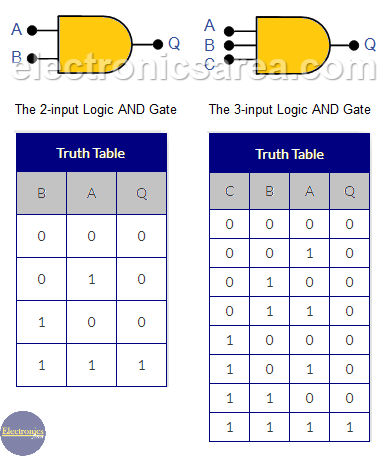2-input and 3-input logic AND Gate – Truth Tables

The 2-input logic AND gate is the most known, although it can have many more inputs (A, B, C, etc.), but it only has one output: Q. The 2-input and the 3-input AND gates have the above truth tables.

It is easy to see that the output Q is “1” (true) when the input A and B are both “1”. In other words, the output Q is “1” when A and B inputs are “1”. The AND gate is represented by Boolean algebra as: Q = A*B or Q = AB. A 3-input logic AND gate implemented with switches is shown in the following diagram.

## How to make a Logic AND Gate implemented using Switches?

To implement an AND logic function using switches, they are placed in series. If all switches are closed the lamp turns on (true / “1”). If one more switches are open lamp turns off (false / “0”)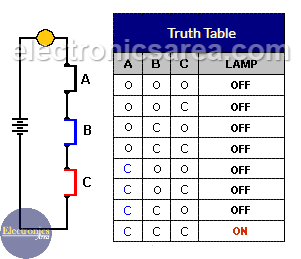Truth table of a 3 switches logic AND gate.  O = Open and C = Closed. Switches are A, B and C.

The AND gate may have many inputs. We can create a multiple input AND gate connecting 2-input AND gates in cascade. The problem connecting AND gates in cascade is that the propagation time (time needed by the signal to travel from the input to the output) increases.

## How to build a 3 input logic AND gate with 2 input AND gates

We can build a 3-input AND gate from 2-input AND gates in cascade as shown in the diagram below.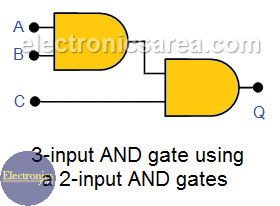The truth table is similar to the one shown above, where switches are used. Using the same method we can implement a 4 or more inputs logic AND gates.

## Multiple-input AND Gate

A 6-input AND Gate can be made using the following circuit diagram: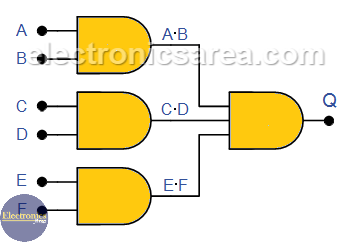###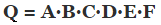This diagram uses one 3-input AND gate and a three 2-input AND gate.

## The TTL 7408 Quad 2-input AND Gate Pinout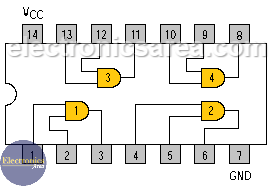• 5
•
•
•
•
•
•
•
•
5
Shares
•
5
Shares
• 5
•
•
•
•
•
•
•